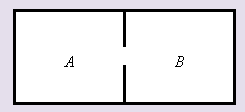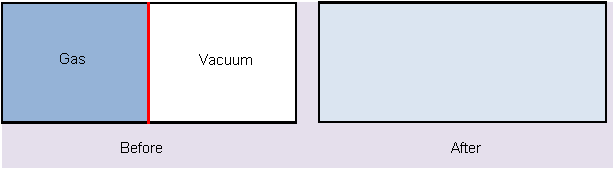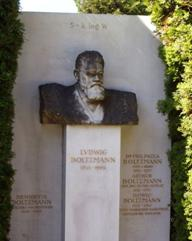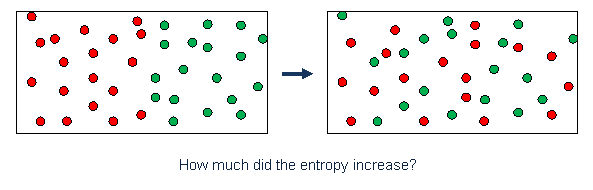$$\require{cancel}$$

# 1.10: Entropy and The Kinetic Theory

•• Contributed by Michael Fowler
• Beams Professor (Physics) at University of Virginia

# Searching for a Molecular Description of Entropy

Clausius introduced entropy as a new thermodynamic variable to measure the “degree of irreversibility” of a process. He believed that his two laws of thermodynamics (conservation of energy and that entropy never decreases) were profound and exact scientific truths, on a par with Newton’s laws of dynamics. Clausius had also made contributions to kinetic theory, publishing work as early as 1857, although unlike Maxwell he did not adopt a statistical point of view—he took all the molecules to have the same speed. (Actually, this work inspired Maxwell’s first investigations of kinetic theory and his discovery of the velocity distribution.) Clausius was the first to attempt a semiquantitative analysis of the effects of molecular collisions (see later).

Obviously, if the kinetic theory is correct, if heat is motion of molecules, the fundamental laws of thermodynamics must be expressible somehow in terms of these molecular motions. In fact, the first law of thermodynamics is easy to understand in this way: heat is just kinetic energy of molecules, and total energy is always conserved, but now instead of having a separate category for heat energy, we can put the molecular kinetic energy together with macroscopic kinetic energy, it’s all ½mv2 with the appropriate masses.

(Note: What about potential energy? For the ideal gas, potential energy terms between molecules are negligible, by definition of ideal gas. Actually, potential energy terms are important for dense gases, and are dominant during phase changes, such as when water boils. The extra energy needed to turn water into steam at the same temperature is called the latent heat. It is simply the energy required to pull the water molecules from each other working against their attraction—in other words, for them to climb the potential energy hill as they move apart. The term “latent heat”— still standard usage— is actually a remnant of the caloric theory: it was thought that this extra heat was caloric fluid that coated the steam molecules to keep them away from each other!)

But how do we formulate entropy in terms of this molecular mechanical model? Boltzmann, at age 22, in 1866, wrote an article “On the Mechanical Meaning of the Second Law of Thermodynamics” in which he claimed to do just that, but his proof only really worked for systems that kept returning to the same configuration, severely limiting its relevance to the real world. Nevertheless, being young and self confident, he thought he’d solved the problem. In 1870, Clausius, unaware of Boltzmann’s work, did what amounted to the same thing. They both claimed to have found a function of the molecular parameters that increased or stayed the same with each collision of particles and which could be identified with the macroscopic entropy. Soon Boltzmann and Clausius were engaged in a spirited argument about who deserved most credit for understanding entropy at the molecular level.

# Enter the Demon

Maxwell was amused by this priority dispute between “these learned Germans” as he termed them: he knew they were both wrong. As he wrote to Rayleigh in December 1870: “if this world is a purely dynamical system and if you accurately reverse the motion of every particle of it at the same instant then all things will happen backwards till the beginning of things the raindrops will collect themselves from the ground and fly up to the clouds &c &c men will see all their friends passing from the grave to the cradle…” because in a purely dynamical system, just particles interacting via forces, the system will run equally well in reverse, so any alleged mechanical measure of entropy that always supposedly increases will in fact decrease in the equally valid mechanical scenario of the system running backwards.But Maxwell had a simpler illustration of why he couldn’t accept always increasing entropy for a mechanical system: his demon. He imagined two compartments containing gas at the same temperature, connected by a tiny hole that would let through one molecule at a time. He knew, of course, from his earlier work, that the molecules on both sides had many different velocities. He imagined a demon doorkeeper, who opened and closed a tiny door, only letting fast molecules one way, slow ones the other way. Gradually one gas would heat up, the other cool, contradicting the second law. But the main point is you don’t really need a demon: over a short enough time period, say, for twenty molecules or so to go each way, a random selection passes through, you won’t get an exact balance of energy transferred, it could go either way.

Maxwell’s point was that the second law is a statistical law: on average over time, the two gases will stay the same temperature, but over short enough times there will be fluctuations. This means there cannot be a mechanical definition of entropy that rigorously increases with time. The whole program of formulating the entropy in terms of mechanical molecular variables is doomed! Evidently, it isn’t at all like the first law.

# Boltzmann Makes the Breakthrough

Despite Maxwell’s deep understanding of the essentially statistical nature of the second law, and Boltzmann’s belief into the 1870’s that there must be a molecular function that always increased and was equivalent to entropy, Boltzmann was the one who finally got it. In 1877, eleven years after his original paper, his senior colleague at the University of Vienna Josef Loschmidt got through to him with the point Maxwell had been making all along, that a mechanical system can run backwards as well as forwards. At that point, it dawned on Boltzmann that entropy can only be a statistical measure, somehow, of the state of the system.

The best way to get a grip on what this statistical measure must be is to take a very simple system, one where we already know the answer from macroscopic thermodynamics, and try to see how the known macroscopic entropy relates to what the molecules are doing. The example we choose is the ideal gas expanding to twice its original volume by removal of a partition:Recall that we established in the last lecture that the change in entropy of n moles of an ideal gas on going from one state (specified uniquely by P, V ) to another to be:

$S(P_2,V_2) - S(P_1, V_1) = C_V ln \dfrac {T_2}{T_1}+ nR \, ln \dfrac{V_2}{V_1} \tag{2.1.1}$

For expansion into a vacuum there will be no temperature change, the molecules just fly through where the partition was, they can’t lose any kinetic energy. The volume doubles, V2 = 2V1, so the entropy increases by

$\Delta S = nR \, ln \, 2 \tag{2.2.2}$

So what’s going on with the molecules? When the volume is doubled, each molecule has twice the space to wander in. The increase in entropy per molecule is simply expressed using Boltzmann’s constant k, and the gas constant R = NAk, where NA is Avogadro’s number (molecules in one mole).

On doubling the volume, there is an entropy increase of k ln 2 per molecule.

So part of the entropy per molecule is evidently Boltzmann’s constant k times the logarithm of how much space the molecule moves around in.

Example 2.1

From equation 2.2.2, one could also increase the entropy by keeping the volume fixed, and increasing the temperature. For a monatomic gas, $$C_V = \dfrac{3}{2} nR$$, doubling the temperature will increase the entropy by $$\dfrac {3}{2} k \, ln \, 2$$ per molecule! Does that relate to “more space”?

Solution

Yes. Remember the lecture on the kinetic theory of gases. To specify the state of the system at a molecular level at some instant of time, we need to say where in the box every molecule is located, plus giving its velocity at that instant.

So the gas fills up two kinds of space: the ordinary space of the box, but also a sphere in velocity space, really a sphere with a fuzzy edge, defined by the Maxwell distribution. It’s a good enough approximation for our purposes to replace this fuzzy sphere with the sharp-edged sphere having radius the root mean square velocity $$v_{rms} = \sqrt { \overline {v^2}}$$. Therefore the volume of the sphere is $$\dfrac {4}{3} \pi v_{rms}^3$$, and this measures how much velocity space a given molecule wanders around in over time and many collisions.

Now, if we heat up the gas, the molecules speed up—which means they spread out to take up more volume in the velocity space. If we double the temperature, since $$\dfrac {1}{2} mv^2 = \dfrac {3}{2} kT$$, $$v_{rms}^2$$, is doubled and the volume of velocity space the gas occupies, measured by the sphere, must go up by a factor of $$2^{3/2}$$.

But $$ln^{3/2} = \dfrac {3}{2} ln \, 2$$, so the entropy increase $$\dfrac {3}{2} k \, ln \, 2$$ on raising the temperature is exactly a measure of the increase in velocity space occupied by the gas, that is, the increase in entropy per molecule is evidently Boltzmann’s constant k times the logarithm of the increase in the velocity space the molecule moves in, exactly analogous to the simple volume increase for the box.

# Epitaph: S = k ln WThe bottom line, then, is that for the ideal gas entropy is a logarithmic measure of how much space a system is spread over, where space is both ordinary space and velocity space. This total space—ordinary space plus velocity space—is called “phase space”. Boltzmann used W as a measure of this total phase space, so the entropy is given by

$S = k \, ln W. \tag{2.2.3}$

This discovery of the molecular meaning of entropy was his greatest achievement; the formula is on his grave.

# But What Are the Units for Measuring W ?

We’ve seen how S = k ln W relates to Clausius’ thermodynamic entropy by looking at the entropy difference between pairs of states, differing first in volume, then in temperature. But to evaluate the entropy for a particular state we need to have a way of explicitly measuring phase space: it would appear that we could move a molecule by an infinitesimal amount and thereby have a different state. Boltzmann himself resolved these difficulties by dividing space and velocity space into little cells, and counting how many different ways N particles could be arranged among these cells, then taking the limit of cell size going to zero. This is of course not very satisfactory, but in fact the ambiguities connected with how precisely to measure the phase space are resolved in quantum mechanics, which provides a natural cell size, essentially that given by the uncertainty principle. Nernst’s theorem, mentioned in the last lecture, that the entropy goes to zero at absolute zero, follows immediately, since at absolute zero any quantum system goes into its lowest energy state, a single state, the ground state: W = 1, so ln W = 0.

Exercise 2.1.1

Recall that an adiabat has equation $$TV^\gamma-1 = constant.$$ Show using the equation above that entropy remains constant: as the spatial volume increases, the velocity space volume shrinks.

# A More Dynamic Picture

We’ve talked interchangeably about the space occupied by the gas, and the space available for one molecule to wander around in. Thinking first about the space occupied by the gas at one instant in time, we mean where the molecules are at that instant, both in the box (position space) and in velocity space. We’re assuming that a single molecule will sample all of this space eventually—in air at room temperature, it collides about ten billion times per second, and each collision is very likely a substantial velocity change. Evidently, the gas as a whole will be passing rapidly through immense numbers of different states: a liter of air has of order 1022 molecules, so the configuration will change of order 1033 times in each second. On a macroscopic level we will be oblivious to all this, the gas will appear to fill the box smoothly, and if we could measure the distribution in velocity space, we would find Maxwell’s distribution, also smoothly filled. Over time, we might imagine the gas wanders through the whole of the phase space volume consistent with its total energy.

The entropy, then, being a logarithmic measure of all the phase space available to the molecules in the system, is a logarithmic measure of the total number of microscopic states of the system, for given macroscopic parameters, say volume and temperature. Boltzmann referred to these many different microscopic states as different “complexions”, all of them appearing the same to the (macroscopic) observer.

# The Removed Partition: What Are the Chances of the Gas Going Back?

We’ve said that any mechanical process could run in reverse, so, really, why shouldn’t the gas in the 2V enclosure happen to find itself at some time in the future all in the left hand half? What are the odds against that, and how does that relate to entropy?

We’ll assume that as a result of the huge number of collisions taking place, the gas is equally likely to be found in any of the different complexions, or molecular configurations—another common word is microstates—corresponding to the macroscopic parameters, the macrostate.

Note

A further mechanism for spreading the gas out in phase space is interaction with the container, which is taken to be at the same temperature, but individual molecular bounces off the walls can exchange energy, over time the exchange will average zero.

Thus the gas is wandering randomly through phase space, and the amount of time it spends with all the molecules in the left-hand half is equal to the corresponding fraction of the total available phase space. This isn’t difficult to estimate: the velocity distribution is irrelevant, we can imagine building up the position distribution at some instant by randomly putting molecules into the container, with a 50% chance of the molecule being in the left-hand half. In fact, we could just pick a state by flipping a coin, and putting the molecule somewhere in the left-hand half if it comes up heads, somewhere on the right if it’s tails, and do this N times (for small N).

Suppose as a preliminary exercise we start with 20 molecules. The chances that we get twenty heads in a row are (1/2)20, or about 1 in 1,000,000. For 40, it’s one in a trillion. For 100 molecules, it’s 1 in 1030.

Example 2.1.2

What is it for a million molecules?

Solution

But a liter of air has of order 1022 molecules! This is never going to happen. So the states having the gas all in the left-hand half of the box are a very tiny fraction of all the states available in the whole box—and the entropy is proportional to the logarithm of the number of available states, so it increases when the partition is removed.

# Demon Fluctuations

Recall in the above discussion of Maxwell’s demon the point was made that for two boxes with a small interconnection, assuming molecules hit the connecting hole randomly from both sides, the energy flow will in fact fluctuate—and clearly so will the number of molecules in one half.

Obviously, then, with our volume 2V, with free access from the left hand half to the right-hand half, the number of molecules in the left-hand half will be constantly fluctuating. The question is: how much? What percentage deviation from exactly 50% of the molecules can we expect to find to the left if we suddenly reinsert the partition and count the molecules?

We can construct a typical state by the coin-toss method described in the previous section, and this is equivalent to a random walk. If you start at the origin, take a step to the right for each molecule placed in the right-hand half, a step to the left for each placed to the left, how far from the origin are you when you’ve placed all the molecules? The details are in my Random Walk Notes, and the bottom line is that for N steps the deviation is of order √N. For example, if the container holds 100 molecules, we can expect a ten percent or so deviation each time we reinsert the partition and count.

But what deviation in density can we expect to see in a container big enough to see, filled with air molecules at normal atmospheric pressure? Let’s take a cube with side 1 millimeter. This contains roughly 1016 molecules. Therefore, the number on the left-hand side fluctuates in time by an amount of order √1016 = 108. This is a pretty large number, but as a fraction of the total number, it’s only 1 part in 108!

The probability of larger fluctuations is incredibly small. The probability of a deviation of m from the average value N/2 is (from Random Walk notes):

$P(m) = Ce^{-\dfrac{2m^2}{N}}. \tag{2.2.4}$

So the probability of a fluctuation of 1 part in 10,000,000, which would be 10√N, is of order $$e^{-200}$$, or about 10-85. Checking the gas every trillionth of a second for the age of the universe wouldn’t get you close to seeing this happen. That is why, on the ordinary human scale, gases seem so smooth and continuous. The kinetic effects do not manifest themselves in observable density or pressure fluctuations—one reason it took so long for the atomic theory to be widely accepted.

# Entropy and “Disorder”

You have probably seen it stated that entropy is a measure of “disorder”. We have defined it above in terms of the amount of phase space the system is moving around in. These are not really different concepts. If we pinned a little label on the molecules, l or r depending on whether it was in the left or the right hand side of the box, the original state had all the labels l. The final state has a random selection of l’s and r’s, very close to equal in numbers.

Another way to say the same thing is to visualize an initial state before removing the partition where the left hand half has “red” molecules, the right hand side “green” molecules (we’re making up these names for visualization purposes, they could be any two gases). If the gases are ideal (or close enough) after removal of the partition both will have twice the volume available, so for each gas the entropy will increase just as for the gas expanding into a vacuum. Thinking about what the state will look like a little later, it is clear that it will be completely disordered, in the sense that the two will be thoroughly mixed, and they are not going to spontaneously separate out to the original state.Figure 2.1.2: Entropy of mixing.

Boltzmann himself commented on order versus disorder with the example of five numbers on a Lotto card. Say the ordered sequence is 1,2,3,4,5. The particular disordered sequence 4,3,1,5,2 is just as unlikely to appear in a random drawing as the ordered sequence, it’s just that there are a lot more disordered sequences. Similarly, in tossing a coin, the exact sequence H, H, T, H, T, T, T, H is no more likely to appear than a straight run of eight heads, but you’re much more likely to have a run totaling four heads and four tails than a run of eight heads, because there are many more such sequences.

# Summary: Entropy, Irreversibility and the Meaning of Never

So, when a system previously restricted to a space is allowed to move into a larger space, the entropy increases in an irreversible way, irreversible meaning that the system will never return to its previous restricted configuration. Statements like this are always to be understood as referring to systems with a large number of particles. Thermodynamics is a science about large numbers of particles. Irreversibility isn’t true if there are only ten molecules. And, for a large number, by “never” we mean it’s extremely unlikely to happen even over a very long period of time, for example a trillion times the age of the universe.

It’s amusing to note that towards the end of the 1800’s there were still many distinguished scientists who refused to believe in atoms, and one of the big reasons why they didn’t was thermodynamics. The laws of thermodynamics had a simple elegance, and vast power in describing a multitude of physical and chemical processes. They were of course based on experiment, but many believed such power and simplicity hinted that the laws were exact. Yet if everything was made of atoms, and Newton’s Laws applied to interactions between atoms, there was a problem. Any dynamical process will run equally well backwards in time. But we always see heat flow from a hot object to a cold object, never the reverse. How could this lack of symmetry come about in an atomic picture? Boltzmann answered this with arguments similar to those given above in the example of the gas initially confined to one half of a box, then allowed to spread out against no resistance. But this meant that ultimately the second law was a statistical statement, not an absolute truth; and, for small enough systems, it isn’t true. But that’s the way it is.

# Everyday Examples of Irreversible Processes

Almost everything that happens in everyday life is, thermodynamically speaking, an irreversible process, and increases total entropy. Consider heat ΔQ flowing by direct thermal contact from a hot body at TH to a cold one at TC. The hot body loses entropy ΔQ/TH , the cold one gains entropy ΔQ/TC , so the net entropy increase as a result is ΔQ/TC - ΔQ/TH, a positive quantity. The heat flow in the Carnot cycle did not increase total entropy, since it was isothermal—the reservoir supplying the heat was at the same temperature as the gas being heated (an idealization—we do need a slight temperature difference for the heat to flow).

Friction, or an inelastic collision such as dropping a ball of clay on the floor, converts kinetic energy to heat—so there is a nonzero ΔQ of heat entering some body, entropy is being generated. Since heat involves random motions of the particles involved, this is sometimes stated as a transformation of ordered energy (a falling ball, with the molecules all moving in the same direction) into disordered motion. Yet another phrasing is that potentially useful energy is being degraded into less useful energy: the falling ball’s kinetic energy could have been utilized in principle by having it caught be a lever arm which could then do work. The slight heat in the floor is far less useful energy, some of it could theoretically be used by a heat engine but the temperature difference to the rest of the floor will be very small, so the engine will be very inefficient!